# CCSS 8.G.1 - video 1 - Translations

##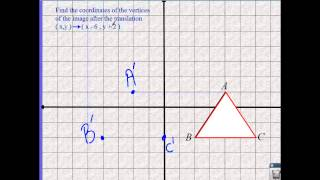By Robert Wirtz

CCSS 8.G.1 - video 1 - Translations# CCSS 8.G.1 - video 1 - Translations

##By Robert Wirtz

CCSS 8.G.1 - video 1 - Translations# CCSS 8.G.1 - video 1 - Translations

##By Robert Wirtz

CCSS 8.G.1 - video 1 - Translations# Geometry - Grade 8 Math Educational Video (8.G.9)

##By Lumos Learning

Using the Lumos Study Programs, parents and educators can reinforce the classroom learning experience for children and help them succeed at school and on the standardized tests. Lumos books, dvd, eLearning and tutoring are used by leading schools, libraries and thousands of parents to supplement classroom learning and improve student achievement in the standardized tests.# 8.g.2

##By SEDL

This mathematics video is from the Common Core State Standards Video Series at http://secc.sedl.org/common_core_videos/# Evaluate composite functions from formulas

##By Khan Academy

Sal solves the following problem: Given that h(x)=3x and g(t)=-2t-2-h(t), find h(g(8)).# Evaluate composite functions from formulas

##By Khan Academy

Sal solves the following problem: Given that h(x)=3x and g(t)=-2t-2-h(t), find h(g(8)).# DEPRECATED Evaluating composite functions

##By Khan Academy

Sal solves the following problem: Given that h(x)=3x and g(t)=-2t-2-h(t), find h(g(8)).# DEPRECATED Evaluating composite functions

##By Khan Academy

Sal solves the following problem: Given that h(x)=3x and g(t)=-2t-2-h(t), find h(g(8)).# Evaluate composite functions from formulas

##By Khan Academy

Sal solves the following problem: Given that h(x)=3x and g(t)=-2t-2-h(t), find h(g(8)).# Evaluate composite functions from formulas

##By Khan Academy

Sal solves the following problem: Given that h(x)=3x and g(t)=-2t-2-h(t), find h(g(8)).# DEPRECATED Evaluating composite functions

##By Khan Academy

Sal solves the following problem: Given that h(x)=3x and g(t)=-2t-2-h(t), find h(g(8)).# DEPRECATED Evaluating composite functions

##By Khan Academy

Sal solves the following problem: Given that h(x)=3x and g(t)=-2t-2-h(t), find h(g(8)).# Distance on the Coordinate Plane 1 (8.G.8)

##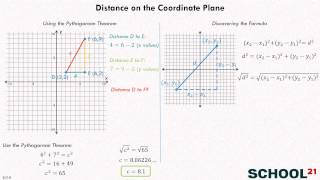By School 21# Understanding Transformations (8.G.1)

##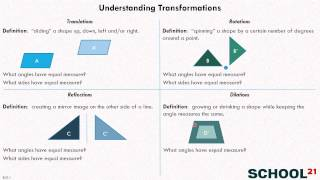By School 21# Similarity 1 (8.G.4)

##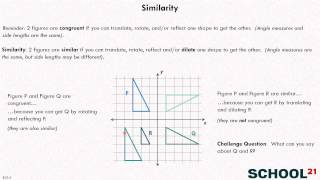By School 21# [8.G.8-1.0] Triangles in Coordinate Plane - Common Core Standard

##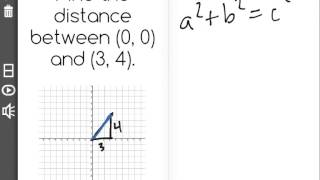By Freckle by Renaissance

Apply the Pythagorean Theorem to find the distance between two points in a coordinate system. Front Row is a free, adaptive, Common Core aligned math program for teachers and students in kindergarten through eighth grade. Front Row allows students to practice math at their own pace - learning advanced concepts when they're ready and receiving remediation when they struggle. Front Row provides teachers with access to a detailed data dashboard and weekly email reports that show which standards are causing students difficulty, what small groups can be formed for interventions, and how their students are progressing in math.# 8.G.4, 5~Transformation of a Line

##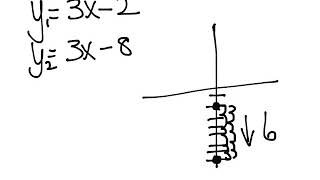By Elizabeth Knutson# Using the Pythagorean Theorem 1 (8.G.7)

##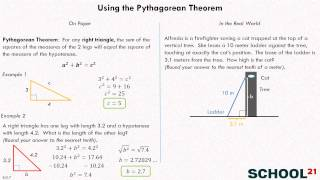By School 21# 8.G.5 - Angle Intro and Vocabulary

##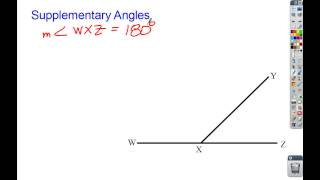By Clarkademy

Angle Intro and Vocabulary